# Markov State Modeling

Basic modeling for P2X7 receptor gating effects

Last updated: August 31st, 2020

## Markov State Modeling Practice¶

#### Purinergic Receptor, P2X7, and its gating mechanism based on ATP concentration¶

Link to the original paper

#### Fig 1: Markov State Model of P2X7¶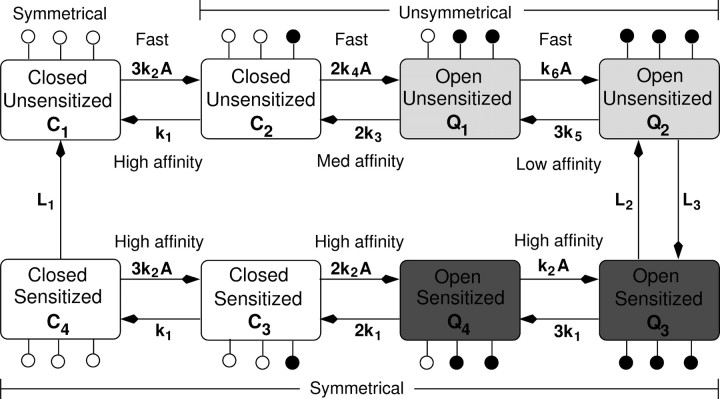#### Following parameters in table are corresponding to the parameters listed in Fig 1 (Markov Map): Ignore "distribution"¶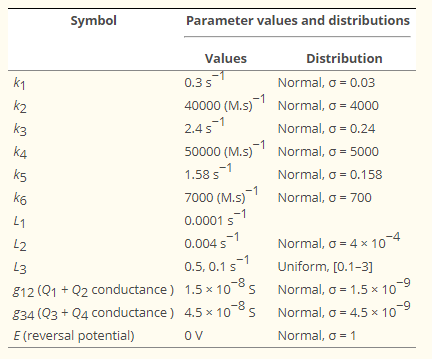Based on Fig 1, you should be able to construct the ordinary differential equations.

For instance,

$\frac{d[C_1]}{dt} = (L_1\times C_4 + k_1\times C_2) - (3k_2\times A\times C_1 + L_4\times C_1)$

• In here $L_4$ is not listed in both table and figure but due to the computation, it is necessary to introduce to stabilize the calculation
• There are two L3 values. You can try either one of them and decide which one works best by comparing the plots from literature given below in Step 4
In :
# Function for integreating ODE and displaying results
import scipy.integrate
from scipy.integrate import odeint
import numpy as np
import matplotlib.pylab as plt
import math
%matplotlib inline


### Step 1: define "ordinary differential equations" based on Fig 1¶

In :
def func(y,t,ATP,t_end):
C1, C2, C3, C4, Q1, Q2, Q3, Q4 = y

### List your parameters such as k1, k2, ....

### ATP switch: ATP is off every 40 seconds.
### Using time variable 't', write out resonating ATP stimulation for 40 seconds on and 40 seconds off

### ODEs: There should be 8 ordinary differential equations for each state starting from C1, C2, ...
dC1dt = L1*C4 + k1*C2       - (3*k2*A + L4)*C1

## list individual ODE in dydt
dydt = []

return dydt


### Step 2: solve ODE¶

In :
## Initial state
y0 = [1,0,0,0,0,0,0,0] ## you need 8 initial states (C1, C2, C3, C4, Q1, Q2, Q3, Q4)

## Time: Ends abount 3 mins after
## Be careful with unit
t_start = 0
t_end   = 120
t_step  = t_end*10
t = scipy.linspace(t_start,t_end,t_step)

## Additional information such as ATP concentration: Test 10, 32, 100 uM.
## Be careful with unit!
## In future, this ATP stimulation would be no longer consistent and we will try.
ATP = 32*10e-6

## Solve!
y = scipy.integrate.odeint() # complete this

<ipython-input-8-247f49d6c430>:9: DeprecationWarning: scipy.linspace is deprecated and will be removed in SciPy 2.0.0, use numpy.linspace instead
t = scipy.linspace(t_start,t_end,t_step)


### Step 3: converting open probability to current¶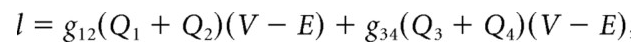• I : inward current
• $Q_{1,2,3,4}$ : open probability from calculated "y"... make sure you understand the index corresponding to these dependent variables and properly extract them to calculate the inward current.
• Tip: if you wonder the structure of "y", type print(np.shape(y)) or print(y) to check what columes and rows indicate
In :
## Parameters
g12 = 1.5e-8
g34 = 4.5e-8
V   = -60e-3
E   = 0

# complete the following expression based on Step3 instruction
I =


### Step 4: Plot and compare them against literature¶

#### Fig 2: Plot from literature¶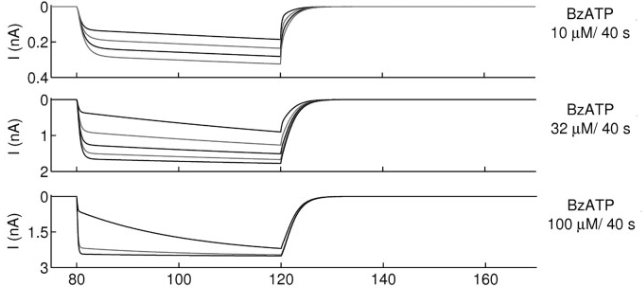In :
plt.figure(figsize=(6,2),dpi=150)
plt.tick_params(labelsize=10,direction='in')
plt.plot(t,I)
plt.tight_layout()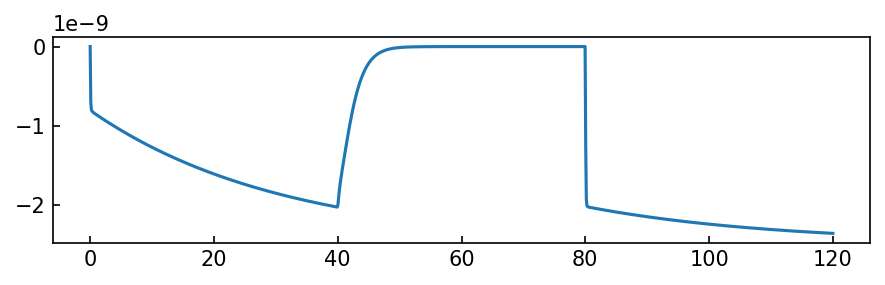In [ ]: